# Java堆结构PriorityQueue完全解析

## PriorityQueue的数据结构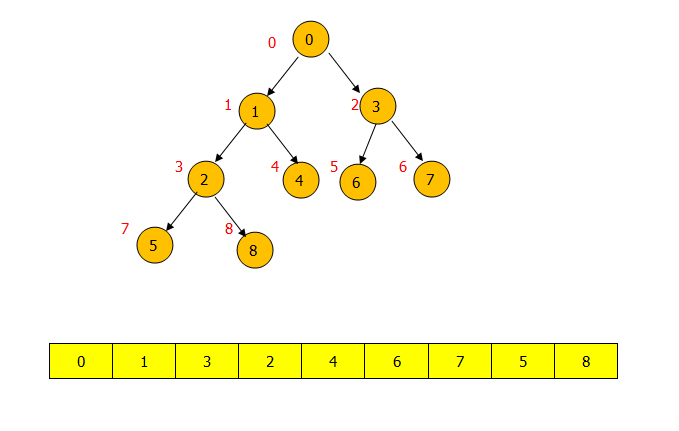PriorityQueue的逻辑结构是一棵完全二叉树，存储结构其实是一个数组。逻辑结构层次遍历的结果刚好是一个数组。

## PriorityQueue的操作

①add(E e) 和 offer(E e) 方法

public boolean add(E e) {
return offer(e);
}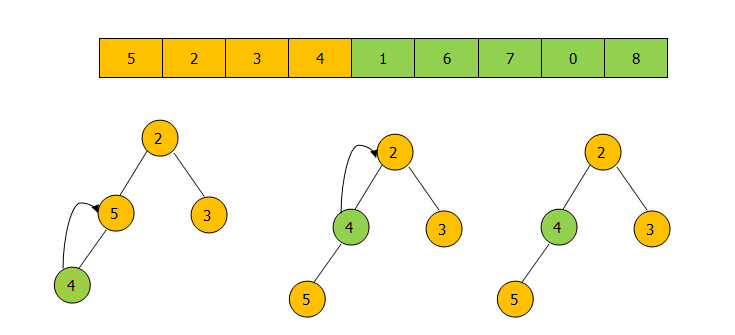public boolean offer(E e) {
if (e == null)
throw new NullPointerException();
//如果压入的元素为null 抛出异常
int i = size;
if (i >= queue.length)
grow(i + 1);
//如果数组的大小不够扩充
size = i + 1;
if (i == 0)
queue = e;
//如果只有一个元素之间放在堆顶
else
siftUp(i, e);
//否则调用siftUp函数从下往上调整堆。
return true;
}

①优先队列中不能存放空元素。
②压入元素后如果数组的大小不够会进行扩充，上面的queue其实就是一个默认初始值为11的数组（也可以赋初始值）。
③offer元素的主要调整逻辑在 siftUp ( i, e )函数中。下面看看 siftUp(i, e) 函数到底是怎样实现的。

private void siftUpComparable(int k, E x) {
Comparable<? super E> key = (Comparable<? super E>) x;
while (k > 0) {
int parent = (k - 1) >>> 1;
Object e = queue[parent];
if (key.compareTo((E) e) >= 0)
break;
queue[k] = e;
k = parent;
}
queue[k] = key;
}


② poll() 和 remove() 方法
poll 方法每次从 PriorityQueue 的头部删除一个节点，也就是从小顶堆的堆顶删除一个节点，而remove（）不仅可以删除头节点而且还可以用 remove(Object o) 来删除堆中的与给定对象相同的最先出现的对象。先看看poll（）方法。下面是poll（）之后堆的操作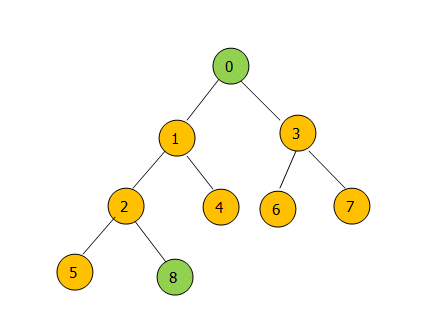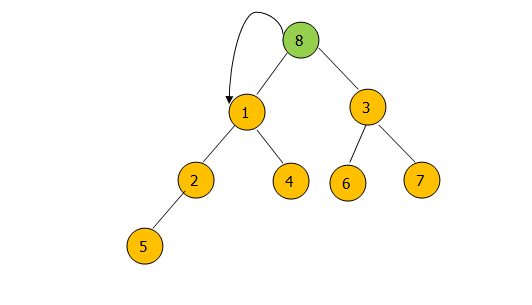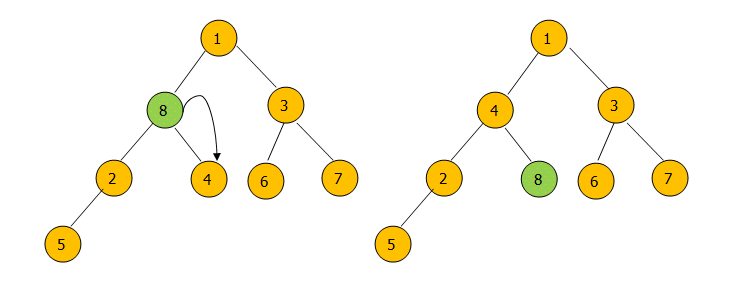poll（）方法的源码

public E poll() {
if (size == 0)
return null;
//如果堆大小为0则返回null
int s = --size;
modCount++;
E result = (E) queue;
E x = (E) queue[s];
queue[s] = null;
//如果堆中只有一个元素直接删除
if (s != 0)
siftDown(0, x);
//否则删除元素后对堆进行调整
return result;
}

private void siftDownComparable(int k, E x) {
Comparable<? super E> key = (Comparable<? super E>)x;
int half = size >>> 1;        // loop while a non-leaf
while (k < half) {
int child = (k << 1) + 1; // assume left child is least
Object c = queue[child];
int right = child + 1;
if (right < size &&
((Comparable<? super E>) c).compareTo((E) queue[right]) > 0)
c = queue[child = right];
if (key.compareTo((E) c) <= 0)
break;
queue[k] = c;
k = child;
}
queue[k] = key;
}

siftDown（）方法就是从堆的第一个元素往下比较，如果比左右孩子节点的最小值小则与最小值交换，交换后继续向下比较，否则停止比较。
remove（4）的过程图：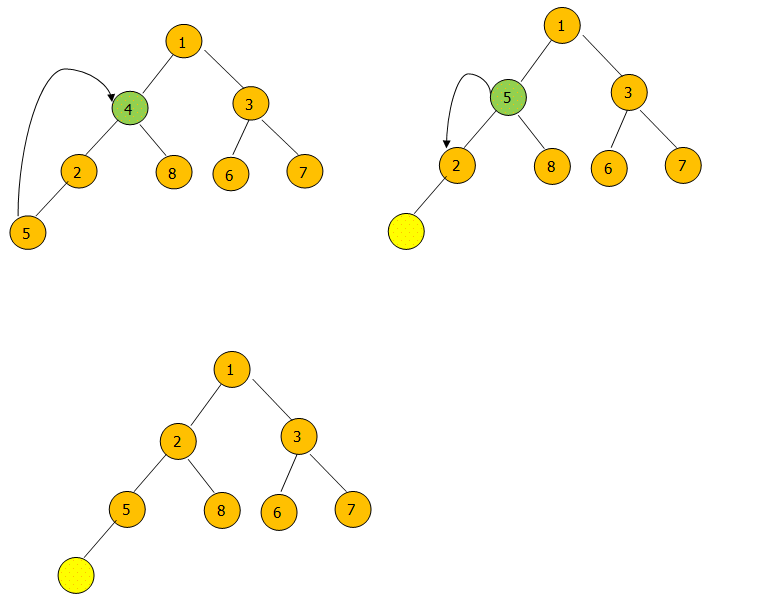remove(Object o)的代码：

 public boolean remove(Object o) {
int i = indexOf(o);
//先在堆中找到o的位置
if (i == -1)
return false;
//如果不存在则返回false。
else {
removeAt(i);
//否则删除数组中第i个位置的值，调整堆。
return true;
}
}

removeAt(int i)的代码

 private E removeAt(int i) {
assert i >= 0 && i < size;
modCount++;
int s = --size;
if (s == i) // removed last element
queue[i] = null;
else {
E moved = (E) queue[s];
queue[s] = null;
siftDown(i, moved);
if (queue[i] == moved) {
siftUp(i, moved);
if (queue[i] != moved)
return moved;
}
}
return null;
}

## 使用PriorityQueue实现大顶堆

PriorityQueue默认是一个小顶堆，然而可以通过传入自定义的Comparator函数来实现大顶堆。如下代码：

 private static final int DEFAULT_INITIAL_CAPACITY = 11;
PriorityQueue<Integer> maxHeap=new PriorityQueue<Integer>(DEFAULT_INITIAL_CAPACITY, new Comparator<Integer>() {
@Override
public int compare(Integer o1, Integer o2) {
return o2-o1;
}
});

02-133万+03-089万+
03-28171
03-181万+
09-021700
03-241万+
03-271104
09-021064
12-1685
©️2020 CSDN 皮肤主题: Age of Ai 设计师:meimeiellie